#### HPage5

Joined Jan 17, 2016
15
HI all,

This forum has become my best friend over the past week. Ive been stuck on a couple problems, posted them here, and had some great advice. So,thanks all.

Anyways, I am stuck again. Mesh and nodal. I really just want a check. My solutions vary slightly form the given solutions and I was curious if I had made a small mistake in my equations/calculations. Maybe someone can see it?

Problem 1--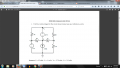I tried a loop method to try and solve this. I started with the bottom right loop (loop1), then the top right (loop2). My assumptions are that V1=12V because it is the first node that the 12V source sees. Also, that V2=6V, because it is the fist node that the 6V source sees.
Equations I built:

loop1: 0.002-V3(2K)-V4(1K)=0
---> 0.002-2000V3-1000V4=0

loop2: V3-12V-V1(1K)-V4(2K)=0
---> V3-12V-12000-2000V4=0
----> V3-2000V4-11988=0

I crunched the system with my Casio and ended up with V3= - 2.99V and V4= 5.99V.

As you can see, my solutions are "correct" (I reckon), with the exception of V3, as it is off from the given - 2.76V.

I tried several other ways, and typically end up with ~3V and ~6V. SO, either Prof. has made an error again, or I have.

Thanks!

#### eeabe

Joined Nov 30, 2013
59
You know that V2=6V because the negative side of the 6V source is at ground potential.

You cannot assume that V1=12V. That would only be true if V3 was 0V. Also, the given answer can't be correct because V1 has to be exactly V3+12V.

I think nodal analysis might be easier on this one, but if you want to use mesh, I'd recommend drawing and labeling all 4 loop currents and writing 4 equations to see how it starts to fall out.

Update: I did nodal analysis and got V3=-(58/21) or about -2.76V, V4=(22/7) or about 3.14V, and of course V2 = 6V and V1 = V3 + 12. I also did a quick check with LTSpice and it looks correct. I found that it quickly reduced down to a system of two equations and two unknowns. I assume it would do the same with mesh.

Last edited:

#### WBahn

Joined Mar 31, 2012
28,182
HI all,

This forum has become my best friend over the past week. Ive been stuck on a couple problems, posted them here, and had some great advice. So,thanks all.

Anyways, I am stuck again. Mesh and nodal. I really just want a check. My solutions vary slightly form the given solutions and I was curious if I had made a small mistake in my equations/calculations. Maybe someone can see it?

Problem 1--

View attachment 99247

I tried a loop method to try and solve this. I started with the bottom right loop (loop1), then the top right (loop2). My assumptions are that V1=12V because it is the first node that the 12V source sees.
Completely invalid reasoning. You get to define the voltage at ONE node and that has already been done for you -- the bottom node is at 0 V. All other node voltages are relative to the voltage of the bottom node.

The 12 V source does not have magical powers to do anything beyond force a voltage relationship between the two nodes it is connected to. It has no knowledge or awareness of which node we have chosen to call 0 V. All the 12 V source does is dictate that Node 1 is 12 V greater than Node 3. This is shown mathematically by

V1 = V3 + 12 V

Also, that V2=6V, because it is the fist node that the 6V source sees.
Right answer, wrong line of reasoning. The 6 V source only dictates that Node 2 is 6 V greater than the bottom node. That means that Node 6 is 6 V, but not because it somehow "sees" Node 2 first. It is because the bottom node has been defined as being 0 V.

Equations I built:

loop1: 0.002-V3(2K)-V4(1K)=0
---> 0.002-2000V3-1000V4=0

loop2: V3-12V-V1(1K)-V4(2K)=0
---> V3-12V-12000-2000V4=0
----> V3-2000V4-11988=0
If you would track your units, you would see that your loop equations make no sense at all.

I'm assuming (which is another problem -- making readers assume things because you aren't being complete) that the 0.002 refers to the current from the current source. I also assume that factors like "2K" refer to various resistances.

You your first loop equation is

0.002 A - V2·(3 kΩ) - V4·(1 kΩ) = 0

Does a voltage multiplied by a resistance yield a current? If not, then how can you add it to (or subtract it from) 0.002 A?

You can't, which means that there is zero point going any bit further with this equation because it is guaranteed to be wrong. Why waste that extra time? Instead, track your units so that you can spot mistakes at the point where they are made so that you can correct them before chasing them down a rabbit hole.

Also, assuming that you are trying to use Ohm's law, to find the current through a resistor, you need to use the voltage ACROSS that resistor. V2 and V4 are the voltages on particular nodes relative to the bottom node -- they are not voltages that appear across resistors.

I tried several other ways, and typically end up with ~3V and ~6V. SO, either Prof. has made an error again, or I have.

Thanks!
The fact that you are ending up with different answers doing it different ways does not bode well for the chances of any one of them being correct (it's always possible, of course).

Instead, leverage that fact that the correctness of the answer can almost always be determined from the answer itself. You simply see if the answers are consistent with the problem. If your answer requires that 10 V appear across a 10 kΩ resistor and also that it have 2 mA of current flowing through it, then you know that your answer is wrong.

There are three primary analysis techniques that you should consider -- node voltage analysis, mesh current analysis, and superposition. I strongly recommend that you use each in turn for the practice. For each one, set up your equations extremely carefully and explicitly. Do not do ANY analysis until ALL of them are set up. This is THE most important part of solving the problem -- if your set up isn't correct, then nothing else matters AND you won't be able to tell until the end when you ask (if you do) whether the answers are consistent with the problem.

Adopt some conventions for how you set up equations and then follow them.

First, always be sure that EVERY symbol you use in any of your equations is clearly defined and annotated on your diagram.
Second, when writing a node equation decide what direction you are going to go around a node and where you are going to start. For instance, always go clockwise and always start with the branch sticking straight up (or the first one clockwise from that position if there isn't one).
Third, when writing a mesh equation, decide what direction your are going to define you mesh currents, in what direction you are going to traverse the mesh, and whether you are going to sum voltage drops or voltage gains as you go.
Fourth, if you are going to use supernodes or supermeshes, then indicate them clearly on the diagram.
Fifth, when doing superposition, draw a different diagram for each case.

#### shteii01

Joined Feb 19, 2010
4,644
Yeah, like others said, you don't understand methodology of Mesh-Current Method.
It is not your job to make assumptions.
YOUR JOB is to follow the current in the mesh. Which, by the way, means that all the voltages at the nodes are USELESS. So your paying attention to them explains how I know that you don't understand Mesh-Current Method of circuit analysis.

Notice that I did not label any of nodes. Why? Because I am using Mesh-Current Method of circuit analysis.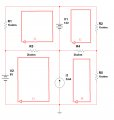#### RBR1317

Joined Nov 13, 2010
706
I also calculated V3= -58/21 and V4= +22/7. Since the answer needs to be in volts it makes good sense to use nodal analysis to find the node voltages. This circuit has two supernodes; V2 forms a supernode (blue) with GND, V1 forms a supernode (orange) with V3, and V4 is an ordinary node. Only two node equations are necessary since one does not write a node equation for the GND node.

Note that all currents at a supernode must sum to zero; however, not all parts of the supernode are at the same voltage. It helps to label the voltage offsets within the supernode since they need to be used to write the node equations. To write a node equation for a supernode, sum all current paths from every node in the supernode to all adjacent nodes not in that supernode, but use the individual node voltages (as labeled).

One supernode equation is shown here to illustrate the technique. Recommend you write and rewrite the node equations for this circuit until you can do it without thinking about it. Then when you come across a complex circuit and fail to see how to apply a clever transformation, you will at least be able to write the node equations without having to think much about it. While mesh analysis and superposition can be useful methods, for me there is no way to apply them without more mental effort than I am usually able to sustain.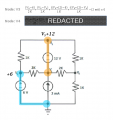#### HPage5

Joined Jan 17, 2016
15
RBR1317, so, to use the supernode you account for everything directly connected to the supernode, not everything in the circuit, correct?

I have analyzed your equation for supernode 1 and I think I get the gist of it. Ive been trying (unsuccessfully) to write an equation for supernode 2. I think the position of it is just confusing me a bit.
Here's an example of one of the things I tried...

supernode2 (blue):
(V4-(V3+12))(1/1k)+V4(1/1k)+(V2-(V3+12))(1/1k)+(V3-V2)(1/2k)=0

I am considering the supernode as a point in the circuit, and trying to account for everything going in out. Am I still thinking incorrectly? My textbook does not go into much detail about the actual use of supernode, it has about 2 sentences saying what a supernode is and thats about it.#### RBR1317

Joined Nov 13, 2010
706
The only reason for identifying the blue supernode is to not write a node equation for it because it's the GND node. That leaves node V4 to write the node equation.

Every term in the node equation represents a current flow expressed as node voltages. Begin each term with the voltage of that node, subtract the adjacent node voltage, and divide by the resistance between those two nodes. Sum these terms for every adjacent node and equate to zero. Current sources must be handled directly. With these conventions, current leaving the node is considered positive.

Now check your node equation for V4. Does every term in the summation begin with V4? V4 has three adjacent nodes; does your summation have three terms? No current source is attached to node V4, no problem. Solve the system of node equations for V3 & V4.

#### WBahn

Joined Mar 31, 2012
28,182
If you understand what a node equation is, then you will have no problem working with a supernode.

A node equation is nothing more than a systematic way of writing KCL, which says that currents leaving a node have to sum to zero.

Well, KCL applies equally well to any black box -- the currents leaving a black box have to sum to zero.

Consider the following: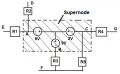KCL says that the sum of the currents leaving the supernode's boundaries is zero. So just write the sum of the currents leaving the supernode's boundaries and set it equal to zero.

$$\frac{V_A \, - V_E}{R_1} \; + \; \frac{V_A \, - V_D}{R_2} \; + \; \frac{V_C \, - V_G}{R_4} \; + \; \frac{V_C \, - V_F}{R_5} \; + \; \frac{V_B \, - V_F}{R_3} \; = \; 0$$

Now, since there are three different nodes at the boundary of the supernode, you need two auxiliary equations to that relate the voltages of these nodes to each other. WHen you define your supernode, you want to do it so that coming up with these auxiliary equations is easy. The best approach is usually to pick one of the boundary nodes and then write each of the others relative to the one you picked. For instance:

Va = Vb + 3 V
Vc = Vb + 7 V

Your supernode has now yielded a total of three equations, one for each boundary node.

That's really all there is to supernodes.

#### shteii01

Joined Feb 19, 2010
4,644
Am I the only one who thinks that this is becoming a waste of time?

Node-Voltage Method.
You have 5 nodes.
One node is reference node.
5-1=4
You must have 4 simultaneous equations to find all the node voltages. One equation for each node, ignore reference node.
Solve the equations and you will have 4 node voltages.
If you need it, use 4 node voltages to find the branch currents by using Ohm's Law.

Mesh-Current Method.
You have 4 meshes.
This means that you must have 4 simultaneous equations. One equation for each mesh current.
Solve the equations, find mesh currents. Use mesh currents to find branch currents.
If you need it, use branch currents to find voltages using Ohm's Law.

#### RBR1317

Joined Nov 13, 2010
706
The best approach is usually to pick one of the boundary nodes and then write each of the others relative to the one you picked.
The only refinement I would make is to label the supernode voltage offsets on the circuit diagram, and use them directly to write the node equation rather than use auxiliary equations. Some people (i.e., me) are less error prone when using a visual method.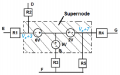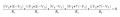#### RBR1317

Joined Nov 13, 2010
706
Am I the only one who thinks that this is becoming a waste of time?
It is only a waste of time if you waste your time on it.

•HPage5

#### WBahn

Joined Mar 31, 2012
28,182
The only problem I have with that is that Vb + 3 is not a voltage since 3 is just a number. It should be Vb + 3 V.

#### WBahn

Joined Mar 31, 2012
28,182
It is only a waste of time if you waste your time on it.
I'm not ready to pack it in on this one yet, either.

•HPage5

#### HPage5

Joined Jan 17, 2016
15
Thanks all, especially WBhan and RBR1317. Not everyone totally understands this stuff. This is an intro class, so, my knowledge of the methods are not yet concrete.

I finally see it though. All terms for Supernode 1 should include variables for V3, because by inspections we can tell that V1=V3+12, and V2=6V. SUpernode 2 needs to be in terms of V4. Supernode 2 is tied to the reference ground so we don't need to worry about it. So, by using supernodes we can cut down the number of equations that we need. My equation for V4 ended up:

(V4-V3)(1/2k)+(V4-(V3+12))(1/1k)+(V4)(1/1k)=0.

simplifying both equations I ended up with:

6V3-3V4+26=0
-3V3+5V4-24=0

solving both:

V3=2.76V
V4=-3.14V

So, I now have both equations, both solutions that I was going for. There is a sign error somewhere, as the signs should be the opposite of what I got. But I think I can figure that out.

Thanks again guys. Really.

#### WBahn

Joined Mar 31, 2012
28,182
Don't put much credence in the answers given in the assignment because you know at first glance that they are wrong.

If V1 = 12 V, then V3 = 0 V, not -2.76 V. Nodes 1 and 3 are connected by a 12 V source, so you KNOW that V1 - V3 MUST be equal to 12 V.